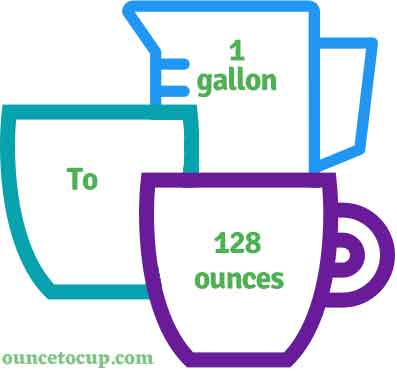# Ounces in a Gallon (gal to oz conversion)

Gallon short form is gal. Are you cooking your favorite dish? The detailed chart in the recipe includes the calculation of the 1 gallon to ounce conversion.  Don't worry; use this calculator to determine how many 1 gallon equals ounces in a minute.  This 1 gal to oz converter gives an exact measurement for any recipe you prepare.

Gallon Value:

gal

Ounce Value:

oz

1 Gallon = 128 Ounce
(1 gal = 128 oz)

Try our auto 1 Gallon to Ounce Calculator (Without Convert Button), Just change the first field value and you got final value.## How many oz is a gal?

We know that the volume value of 1 gal is equal to 128 oz. If you want to convert 1 gal to an equal number of oz, just multiply the volume value by 128 or divide the value by 29.5735. Hence, 1 gallon is equal to 128 oz.

The Answer is: 1 US Gallons = 128 US Fluid Ounce

1 gal = 128 oz

Many of them try to search or find an answer for what is 1 gallons in oz? So, we’ll start with 1 gal to oz conversion to know how big is 1 gal.

## How To Calculate 1 gal to oz?

To calculate 1 gallons to an equal number of ounce, simply follow the steps below.

Gallons to Fliud Ounces formula is:

Ounce = Gallon * 128

Assume that we are finding out how many oz were found in 1 fl gal of water, multiply by 128 to get the result.

Applying to Formula: oz = 1 gal * 128 = 128 oz.

## How To Convert gal to oz?

• To convert gallons to oz,
• Simply multiply the gallon value by 128.
• Applying to the formula, oz = 1 gallons * 128 [1x128].
• Hence, 1 gallons is equal to 128 oz.

## Some quick table references for ounces to gallon conversions:

Gallon [gal]Ounces [oz]
1 gal128 oz
2 gal256 oz
3 gal384 oz
4 gal512 oz
5 gal640 oz
6 gal768 oz
7 gal896 oz
8 gal1024 oz
9 gal1152 oz
10 gal1280 oz
11 gal1408 oz
12 gal1536 oz
13 gal1664 oz
14 gal1792 oz
15 gal1920 oz

## Reverse Calculation: How many gallons are in a oz?

• To convert 1 oz to gal,
• Simply divide the 1 oz by 128.
• Then, applying the formula, gal = 1 oz / 128 [1/128 = 0.0078125].
• Hence, 1 oz is equal to 0.0078125 gal.